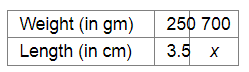# The amount of extension in an elastic spring varies directly with the weight hung on it.`
Question:

The amount of extension in an elastic spring varies directly with the weight hung on it. If a weight of 250 gm produces an extension of 3.5 cm, find the extension produced by the weight of 700 gm.

Solution:

Let x cm be the extension produced by the weight of 700 gm.Since the amount of extension in an elastic spring varies and the weight hung on it is in direct variation, we have :

$\frac{250}{700}=\frac{3.5}{x}$

$\Rightarrow x \times 250=3.5 \times 700$

$\Rightarrow x=\frac{3.5 \times 700}{250}$

$=\frac{2450}{250}$

$=9.8$

Thus, the required extension will be $9.8 \mathrm{~cm}$.